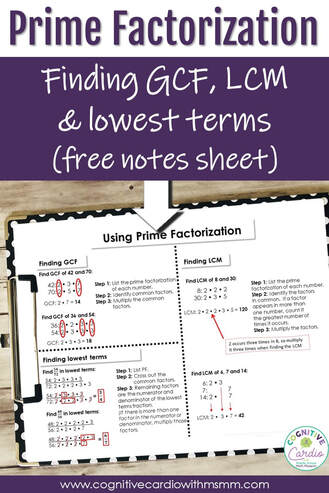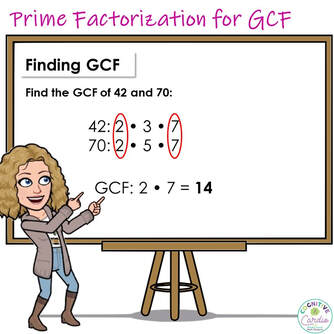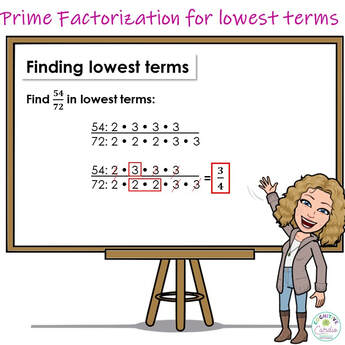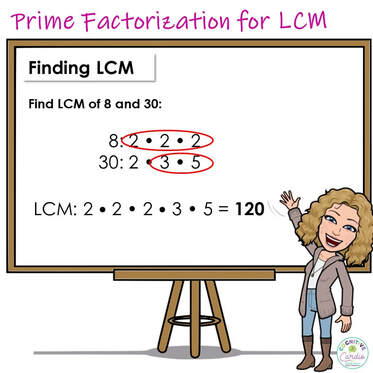# Three Ways to Use Prime Factorization in Middle School MathLoving Prime Factorization
​Is it weird that I love prime factorization??

Every year of teaching math, I have come to appreciate prime factorization more and more! Maybe it’s because when I was a student (forever ago!), I didn’t learn how to use prime factorization to find greatest common factors, least common multiples or to reduce fractions. (I will admit to the possibility that I learned and forgot….but I truly think I didn’t learn it!). In addition to missing this information as a student, I didn’t find it in math teacher manuals until I’d been teaching for more than 20 years.

I’ll share why I love it so much by explaining three ways to use prime factorization: to find GCF, LCM, and lowest terms for fractions.How to Find Greatest Common Factor With Prime Factorization
1) First, find the prime factorization of each number.
Using the example in the image:

• the prime factorization of 42 is 2 x 3 x 7
• the prime factorization of 70 is 2 x 5 x 7

2)Next, identify the factors the numbers have in common

• 24 and 70 both have a 2 and a 7

3) Last, multiply the factors that are in common

• 2 x 7 = 14, so GCF is 14

Why do I like this method? I like using prime factorization to find greatest common factor because when my students use the ‘listing method, they often miss factors of some numbers; and when they miss factors, I swear they always end up missing the GCF.

​Using prime factorization, they DON’T miss these factors, so they’re more successful in identifying the GCF.

​Love it!How to Find Lowest Terms Using Prime Factorization:
In past years, when students reduced fractions, they often chose ANY factor to divide by, (unless they were forced to find the GCF). Then, they would reduce and reduce again, and sometimes they still didn’t reach lowest terms.

For example, some students would take 54/72 and divide by 2 to get 27/36. Then they might divide by 3 to reach 9/12. Some might stop here and never reach 3/4 as the lowest terms.

To find lowest terms using prime factorization:
1) First, find the prime factorization of each number.
2) Next, cross out the factors that are in common.

• In the image example, 54 and 72 have one 2 and two 3s in common, so those factors are crossed out

3) The remaining factors are the numerator and denominator of the reduced fraction

• If more than one factor remains in either the numerator or denominator, they should be multipliedHow to find Least Common Multiple with Prime Factorization
Using prime factorization to find the least common multiple is fantastic! Listing multiples can be pretty tedious (though it does reinforce multiplication facts), and although finding the prime factorization might be difficult for students to begin with, it will eventually be quicker than listing multiples.

Once I started talked about prime factorization a lot in math class, and thought about it aloud so the students could hear my thought process in breaking down numbers, my students started to find prime factorizations much more quickly than students in the past.

To find LCM with prime factorization:
1) First, find the prime factorization of each number.
2) Next, identify the different factors of each number.

• If a factor is found in both numbers, count it the greatest number of times it appears in either number. For example, in the prime factorizations of 8 and 30 (image above), 2 occurs three times in 8 and only once in 30, so it is used three times in the LCM calculation.

A 2nd Example: Find the LCM of 6, 7, and 14
1) Find the prime factorization of each number.

• 6: 2 x 3
• 7: 7
• 14: 2 x 7

2) Identify the different factors of each number.
The factors 2, 3, and 7 occur once at most, so they are each multiplied once to find the LCM of 42.

Other Prime Factorization Benefits
Besides helping students to find GCF and LCM and reduce fractions more quickly, I love the fact that using prime factorization for these concepts helps students develop a better understanding of relationships between numbers….I see and hear this awareness developing.

Here’s a great benefit – students like it! While some students are comfortable with ways they’ve learned in previous years and are hesitant to use prime factorization, other students have actually come to me during our study period to double check how to use prime factorization in these ways, because they LIKE it and think it’s cool!

I’ve created a note sheet for my students to keep in their notebooks so they can refer to it throughout the year. Feel free to download and use it!
Use the Ladder Method to Find Prime Factorizations
Of course, I also love how the ladder method can be used to find prime factorizations, as well as GCF, LCM, and lowest terms fractions. You can read about that in this post!

I hope you enjoy prime factorization! Are there any other ways you use prime factorization with your students?

Other Resources for GCF, LCM, and Prime Factorization Instruction and Practice
If you’re looking for other resources to help your students practice with GCF, LCM or prime factorization, I have several in my TeachersPayTeachers shop – some print and some digital:

## EllieWelcome to Cognitive Cardio Math! I’m Ellie, a wife, mom, grandma, and dog ‘mom,’ and I’ve spent just about my whole life in school! With nearly 30 years in education, I’ve taught:

• All subject areas in 4th and 5th grades
• Math, ELA, and science in 6th grade (middle school)

I’ve been creating resources for teachers since 2012 and have worked in the elearning industry for about five years as well!